Levels »

# Don’t Get Poisoned

### The Hole, Elevation: -1042.4m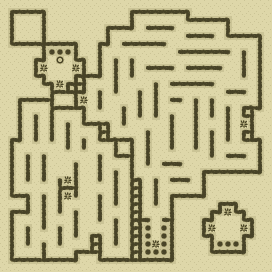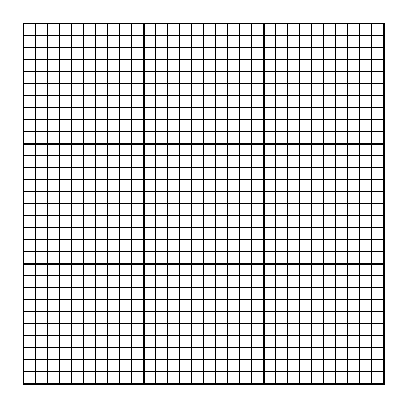### Items

•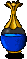3 Clear Blue Potions (22, 10) - (3, 30) - (14, 30)
•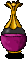Pale Violet Potion (19, 1)

### Monsters

•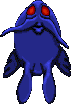12 Greater Nightmares (Random rate: 1) (1, 1) - (19, 1) - (19, 1) - (3, 3) - (15, 9) - (12, 10) - (22, 14) - (14, 19) - (23, 19) - (18, 28) - (2, 30) - (3, 30)
•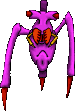48 Venemous Skitters (3, 1) - (16, 1) - (18, 1) - (20, 1) - (19, 2) - (21, 2) - (1, 3) - (16, 3) - (20, 3) - (15, 5) - (20, 6) - (28, 8) - (12, 10) - (14, 10) - (21, 10) - (3, 12) - (12, 12) - (26, 12) - (2, 14) - (17, 14) - (8, 15) - (26, 15) - (23, 18) - (9, 19) - (23, 19) - (24, 19) - (30, 19) - (3, 20) - (14, 20) - (16, 20) - (23, 20) - (14, 21) - (5, 22) - (17, 22) - (19, 22) - (5, 24) - (3, 25) - (12, 25) - (1, 26) - (3, 26) - (12, 27) - (3, 28) - (1, 29) - (3, 29) - (3, 29) - (8, 29) - (14, 29) - (1, 30)
•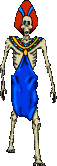Ghasts (Random rate: 6)

### Locations

• Save rune(6, 6)

### Teleporters

• (4, 7) Teleporter to (7, 21)
• (8, 7) Teleporter to (7, 23)
• (6, 9) Teleporter to (27, 25)
• (9, 11) Teleporter to (25, 27)
• (29, 14) Teleporter to (29, 27)
• (7, 21) Teleporter to (4, 7)
• (7, 23) Teleporter to (8, 7)
• (27, 25) Teleporter to (6, 9)
• (25, 27) Teleporter to (9, 11)
• (29, 27) Teleporter to (29, 14)